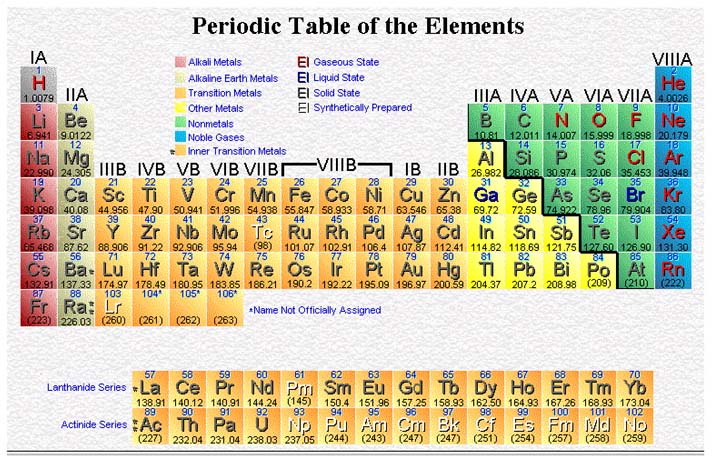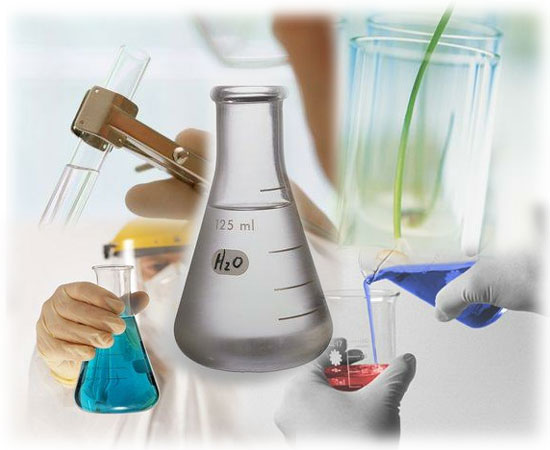CHEMISTRY Pre-AP Unit 12: Gas LawsGoal: The student should be able to:

1. State the relationship between the pressure of a gas and volume of a gas.

2. Write the equation for and apply Boyle's Law in solving problems and state the conditions under which Boyle's Law can be used.

3. State the relationship between the temperature of a gas and the volume of a gas.

4. Write the equation for and apply Charles' Law in solving problems and state the conditions under which Charles' Law can be used.

5. State the relationship between the temperature of a gas and the pressure of a gas.

6. Write the equation for and apply Gay-Lussac’s Law in solving problems and state the conditions under which the Gay-Lussac Law can be used.

7. Correct the pressure of a certain volume of a gas when it is collected over water or when it is known to be saturated with water vapor.

8. Apply the combined gas lawin working problems including stoichiometry at nonstandard conditions of temperature and pressure.

9. State the effect of pressure and temperature on the density of a gas.

10. Correct the density of gas to STP given the volume, temperature and pressure.

11. Explain and apply the Ideal Gas Law.

12. Use the Ideal Gas Law to compute molecular mass.

13. List the properties of real gases, which lead to deviations from the model of an ideal gas and thus deviation from the Ideal gas- laws.

Activities:

February 23: Discuss Unit XI Test, Begin Lecture Gas Laws

February 26: Lab: Melting and Freezing Points

February 27: Discuss problems, Finish Lecture Gas Laws

February 28: Discuss problems, Lecture Ideal Gas Law

March 1 : Discuss problems, Lecture Gas Stoichiometry

March 2 : Lab: Boyle's Law and Gay-Lussac's Law

March 5 : Lab: Boyle's Law and Gay-Lussac's Law

March 6 : Discuss problems, Review for test

March 7 : Unit XII Test

Homework:

February 23: Practice Problems 1-13, 19-23

February 26: Lab report due March 5

February 27: Practice Problems 24-33

February 28: Practice Problems 41-50

March 1 : Practice Problems 56-64, Prelab for Boyle's Law and Gay-Lussac's Law

March 2 : Finish lab report on Melting Points

March 5 : Lab report due Feb 22

March 6 : Discuss problems, Review for test.

Lecture & Lab Materials (uploaded once per week):

Gas Law Problems